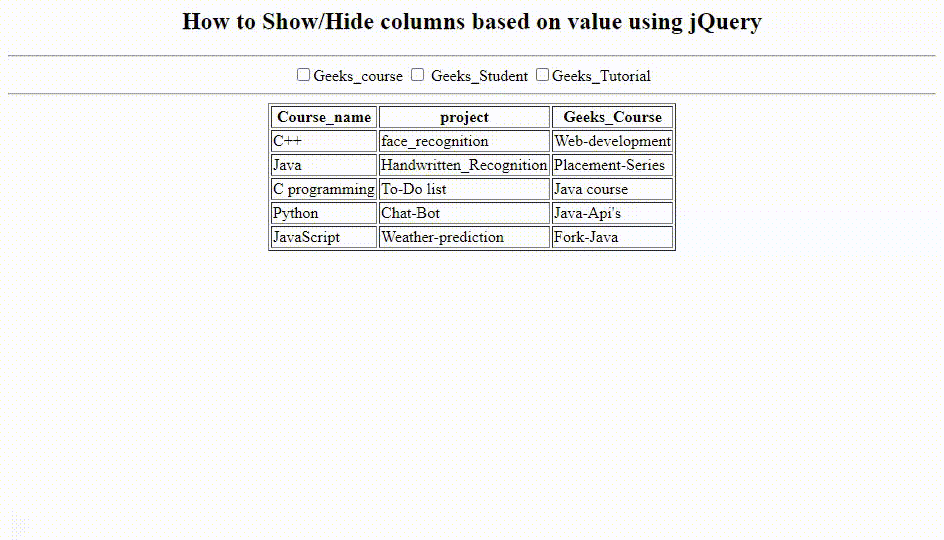Open In App

# How to hide the checkbox based on value in jQuery ?

The checkbox are used to set the content according to the user which he wants to see at the interface. User also can set the multiple choices according to his wish.

Syntax:

```\$('input[name=foo]').attr('checked', false);
```

In the above syntax, it returns the value of the checked or unchecked checkbox being assigned to the table box which the user selects. If the user want to see the particular content in the table box, then the user can select the checkbox associated with the column textbox which he wants.

Example: Below example illustrates the approach. It returns the value of the checkbox assigned to the table box or column using a function click(). Whenever the checkbox is checked or unchecked, it assigns the respective return value into the table box.

## html

 ```<``html``>`` ` `<``head``>``    ``<``center``>``        ``<``h2``>``            ``How to Show/Hide checkbox based ``            ``on value using jQuery``        ```` ` `        ``<``hr` `/>``        ``<``input` `type``=``"checkbox"` `name``=``"Course"``>Geeks_course``        ``<``input` `type``=``"checkbox"` `name``=``"student"``> Geeks_Student``        ``<``input` `type``=``"checkbox"` `name``=``"Tutorial"``>Geeks_Tutorial``        ``<``hr` `/>`` ` `        ``<``script` `src``=``"https://ajax.googleapis.com/ajax/libs/jquery/3.5.1/jquery.min.js"``>``        ````         ` `        ``<``script``>``            ``\$("input:checkbox").attr("checked", ``                    ``false).click(function () {``                ``var shcolumn = "." + \$(this).attr("name");``                ``\$(shcolumn).toggle();`` ` `            ``});``        ```` ` ``` ` `<``body``>``    ``<``table` `border``=``"1"``>``        ``<``tr``>``            ``<``th` `class``=``"Course"``>Course_name``            ``<``th` `class``=``"student"``>project``            ``<``th` `class``=``"Tutorial"``>Geeks_Course``        ````        ``<``tbody``>``            ``<``tr``>``                ``<``td` `class``=``"Course"``>C++``                ``<``td` `class``=``"student"``>face_recognition``                ``<``td` `class``=``"Tutorial"``>Web-development``            ````            ``<``tr``>``                ``<``td` `class``=``"Course"``>Java``                ``<``td` `class``=``"student"``>Handwritten_Recognition``                ``<``td` `class``=``"Tutorial"``>Placement-Series`` ` `            ````            ``<``tr``>``                ``<``td` `class``=``"Course"``>C programming``                ``<``td` `class``=``"student"``>To-Do list``                ``<``td` `class``=``"Tutorial"``>Java course`` ` `            ````            ``<``tr``>``                ``<``td` `class``=``"Course"``>Python``                ``<``td` `class``=``"student"``>Chat-Bot``                ``<``td` `class``=``"Tutorial"``>Java-Api's`` ` `            ````            ``<``tr``>``                ``<``td` `class``=``"Course"``>JavaScript``                ``<``td` `class``=``"student"``>Weather-prediction``                ``<``td` `class``=``"Tutorial"``>Fork-Java`` ` `            ````        ````    `````````` ` ``

Output: